##### Basic Math & Pre-Algebra All-in-One For Dummies (+ Chapter Quizzes Online)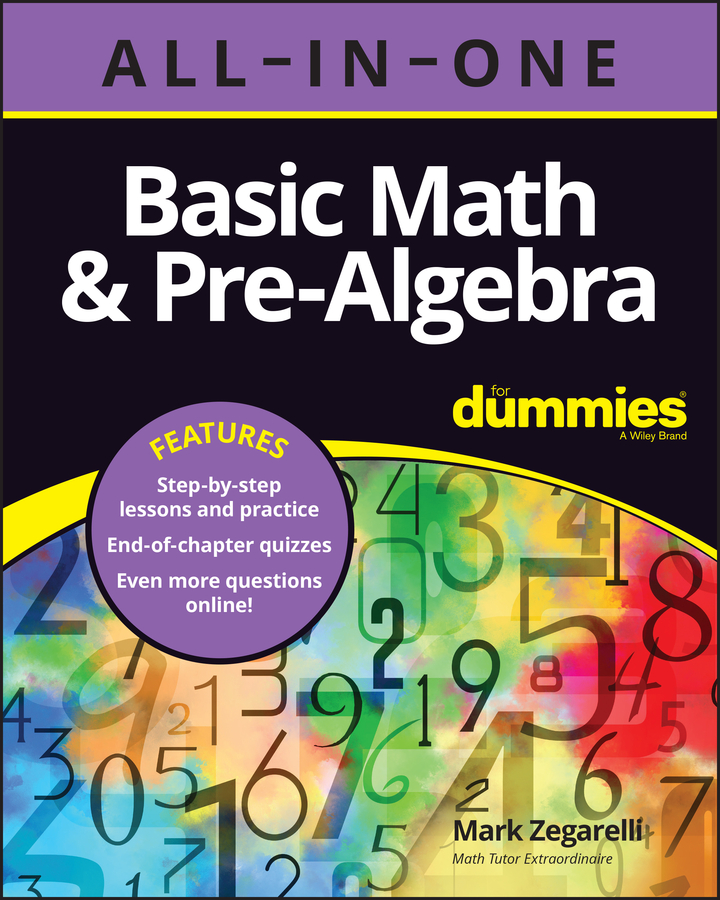When you add two fractions that have the same denominator (bottom number), you simply add the numerators (top numbers) together and leave the denominator unchanged.

For example, consider the following problem: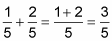As you can see, to add these two fractions, you add the numerators (1 + 2) and keep the denominator (5).

Why does this work? Well, you can think about fractions as pieces of cake. The denominator in this case tells you that the entire cake has been cut into five pieces. So when you add 1/5 + 2/5, you’re really adding one piece plus two pieces. The answer, of course, is three pieces — that is, 3/5.

Even if you have to add more than two fractions, as long as the denominators are all the same, you just add the numerators and leave the denominator unchanged: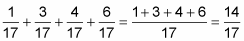Sometimes, when you add fractions with the same denominator, you may have to reduce it to lowest terms. Take this problem, for example: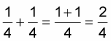The numerator and the denominator are both even, so you know they can be reduced: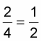In other cases, the sum of two proper fractions is an improper fraction. You get a numerator that’s larger than the denominator when the two fractions add up to more than 1, as in this case: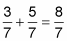If you have more work to do with this fraction, leave it as an improper fraction so that it’s easier to work with. But if this is your final answer, you may need to turn it into a mixed number: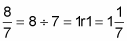When two fractions have the same numerator, don’t add them by adding the denominators and leaving the numerator unchanged.